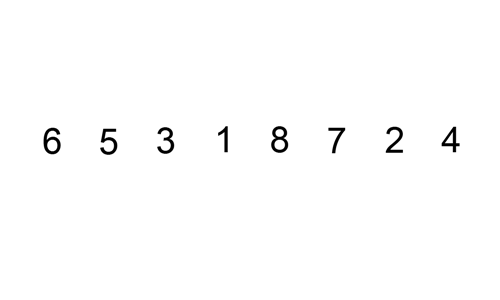# Swapping ints 2We know that the insertion sort algorithm has a worst-case running time of $O(n^{2})$ in general case. What if we could bound the algorithm for specific instance of the problem. One way to do that is by checking the number of swap operations needed by insertion algorithm to sort a list.

In the permutation of $a_{1}\cdots a_{n}$ of $n$ distinct elements the list inversion are pair of elements $(a_{i},a_{j})$ such that $i and $a_{i} > a_{j}$. What is the worst-case running time if the inputs are restricted to permutations of $1\cdots n$ with at most $n$ inversions?

×